# Lecture 27: Error of quadrature¶

Last lecture we observed the following:

1. The right-hand rule had error $O(n^{-1})$ for $n$ sample point
2. The trapezium rule had error $O(n^{-2})$ for $n$ sample point
3. The trapezium rule had error $O(n^{-\alpha})$ for all choices of $\alpha \geq 0$ for smooth, periodic functions

This lecture we set out to prove these results.

## Right-hand rule convergence¶

Recall that the right-hand rule is defined by, for $x_k \triangleq kh$ and $h \triangleq 1/n$

$$\int_0^1 f(x) dx \approx h \sum_{k=1}^n f(x_k) = \sum_{k=1}^n \int_{x_{k-1}}^{x_k} (f(x) - f(x_k) ) dx$$

About the only tool available to analyse integrals is integration by parts:

\begin{align*} \int_a^b u(x) v'(x) dx &= [u(x) v(x)]_a^b - \int_a^b u'(x) v(x) \cr & = u(b) v(b) - u(a)v(a) - \int_a^b u'(x) v(x) \end{align*}

Consider the error in the first panel. Taking $u(x) = f(x)$ and $v(x) = x$ in the integration by parts formula yields:

\begin{align*} \int_0^h (f(x) - f(h)) dx = [(f(x) - f(h)) x]_0^h - \int_0^h f'(x) x dx \cr = - \int_0^h f'(x) x dx \end{align*}

Assume that $f'(x)$ is bounded in $[0,1]$, and define

$$M= \sup_{x \in [0,1]} |f'(x)|$$

We then get a bound on the error:

\begin{align*} \left|\int_0^h (f(x) - f(h)) dx\right| &= \left|\int_0^h f'(x) x dx\right| \leq \int_0^h |f'(x)| x dx \leq M \int_0^h x dx \cr &\leq {M h^2 \over 2} \end{align*}




This formula caries over to every other panel (by the same argument):

$$\left|\int_{x_{k-1}}^{x_k} (f(x) - f(x_k)) dx \right| \leq {M h^2 \over 2}$$

Thus we have

\begin{align*} \left\|\int_0^1 f(x) dx - h \sum_{k=1}^n f(x_k)\right\| &= \left\|\sum_{k=1}^n \int_{x_{k-1}}^{x_k} (f(x) - f(x_k) ) dx\right\| \cr &\leq \sum_{k=1}^n \left\|\int_{x_{k-1}}^{x_k} (f(x) - f(x_k) ) \right\| dx &\leq \sum_{k=1}^n {M h^2 \over 2} = {M h^2 n \over 2} = {M \over 2 n} = O(n^{-1}) \end{align*}

Thus we have proven that the right-hand rule converges like $O(n^{-1})$.

## Trapezium rule observed convergence, revisited¶

Recall the trapezium rule, here implemented for general intervals $[a,b]$:

In :
using PyPlot

function trap(f,a,b,n)
h=(b-a)/n
x=linspace(a,b,n+1)

v=f(x)
h/2*v+sum(v[2:end-1]*h)+h/2*v[end]
end

trap(f,n)=trap(f,0.,1.,n);


Consider integration of the following simple function:

In :
f=x->exp(x).*cos(x)

g=linspace(0.,1.,1000)
plot(g,f(g));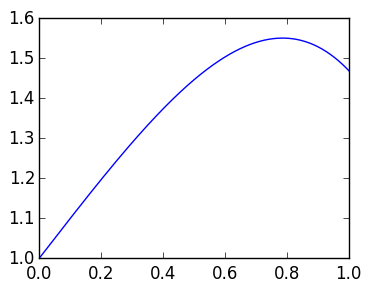As in last lecture, we can compare the error of trap to the exact integral, approximated by quadgk.

In :
ex=quadgk(f,0.,1.)

ns=round(Int,logspace(1,5,100))   # integers spaced logarithmically apart
errT=zeros(length(ns))
errπ=zeros(length(ns))

for k=1:length(ns)
n=ns[k]
errT[k]=abs(trap(f,n-1)-ex    )   # error in non-periodic
errπ[k]=abs(trap(θ->cos(cos(θ-0.1)),0.,2π,n-1)-exπ    )  # error in periodic
end


We see that for non-periodic functions we observe $O(n^{-2})$ convergence but for periodic functions we converge much faster:

In :
loglog(ns,errT)   # blue curve
loglog(ns,errπ)   # green curve
loglog(ns,(1.0ns).^(-2))   # red curve, with same slope as blue curve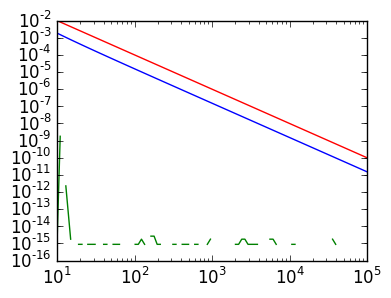Out:
1-element Array{Any,1}:
PyObject <matplotlib.lines.Line2D object at 0x304c5ed90>

The defining property of smooth, periodic functions is that the function and all its derivatives match at 0 and 2π. Now consider a function where only some of he derivatives match:

In :
h=x->10f(x).*x.^2.*(1-x).^2

plot(g,h(g));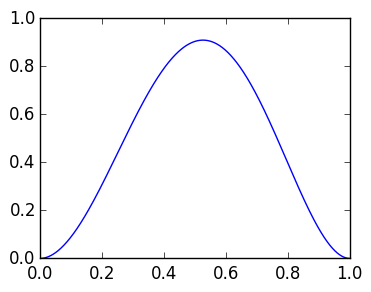We see here that the convergence rate has converged to $O(n^{-4})$:

In :
exf=quadgk(f,0.,1.)

ns=round(Int,logspace(1,4,100))
errf=zeros(length(ns))
errh=zeros(length(ns))

for k=1:length(ns)
n=ns[k]
errf[k]=abs(trap(f,n-1)-exf    )
errh[k]=abs(trap(h,n-1)-exh    )
end

loglog(ns,errf)     # blue curve
loglog(ns,1./ns.^2) # green curve
loglog(ns,errh)     # red curve
loglog(ns,1./ns.^4) # aqua curve;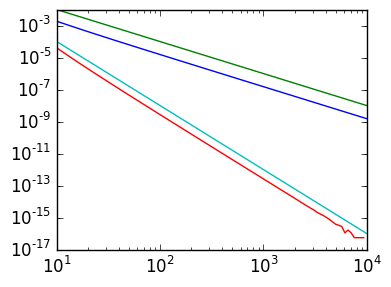The conclusion is that derivatives at the endpoints dictate the convergence rate of the Trapezium rule.

# Bernoulli Polynomial¶

To prove this, we need to be clever about our choice of functions when we integrate by parts. Define the first three Bernoulli Polynomials by

$$B_0(x) = 1, B_1(x) = x-{1 \over 2}, B_2(x) = x^2 -x + {1 \over 6}$$

as depticted here:

In :
g=linspace(0.,1.,1000)
B0=x->ones(x)
B1=x->x-1/2
B2=x->x.^2-x+1/6
plot(g,B0(g))
plot(g,B1(g))
plot(g,B2(g));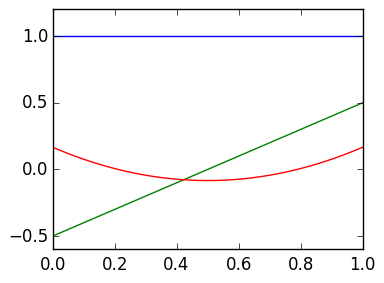These polynomials satisfy two important properties:

1. $B_k'(x) = k B_{k-1}(x)$, just like monomials $x^k$
2. $B_2(-1) = B_2(1) = {1 \over 6} We Thus consider integration by parts with the Trapezium rule. Recall the Trapezium rule: $$\int_0^1 f(x) dx \approx h\left({f(x_0) \over 2} + \sum_{k=1}^{n-1} f(x_k) + {f(x_n) \over 2} \right) = \sum_{k=1}^n \int_0^1 \left(f(x_{k-1}) + (x-x_{k-1}) {f(x_k) - f(x_{k-1}) \over h}\right) dx$$ As in the right-hand rule, we begin with the error in the first panel: $$\int_0^h \left[f(x) - \left(f(0) + x {f(h) - f(0) \over h}\right) \right] dx.$$ Taking $$u(x) = f(x) - \left(f(0) + x {f(h) - f(0) \over h}\right)$$ and$v(x) = h B_1(x/h)$(so that$v'(x) = 1$) in the integration by parts formula: $$\int_0^h \left[f(x) - \left(f(0) + x {f(h) - f(0) \over h}\right) \right] dx = [u(x) v(x)]_0^h - \int_0^h u(x) v(x) dx = - h \int_0^h \left[f'(x) - {f(h) - f(0) \over h}\right] B_1(x/h) dx.$$ Here we used that$u(0) = u(h) = 0$to kill of the first terms. Now take$u(x) = f'(x) - {f(h) - f(0) \over h}$and$v(x) = h {B_2(x/h) \over 2}$(so that$v'(x) = B_1(x/h)): \begin{align*} - h \int_0^h \left[f(x) - {f(h) - f(0) \over h}\right] B_1(x/h) dx &= -{h^2 \over 2}\left[\left(f'(h) - {f(h) - f(0) \over h}\right) B_1(1) - \left(f'(0) - {f(h) - f(0) \over h}\right) B_1(-1)\right] \cr & \qquad +{h^2 \over 2} \int_0^h f''(x) B_2(x/h) dx \cr & = - {f'(h) - f'(0) \over 12} h^2 +{h^2 \over 2} \int_0^h f''(x) B_2(x/h) dx \end{align*} By the same logic, we have in each panel $$\int_{x_{k-1}}^{x_k} \left[f(x) - \left(f(x_{k-1}) + x {f(x_k) - f(x_{k-1}) \over h}\right) \right] dx = - {f'(x_k) - f'(x_{k-1}) \over 12} h^2 +{h^2 \over 2} \int_{x_{k-1}}^{x_k} f''(x) B_2((x-x_{k-1})/h) dx$$ Summing over every panel, and using the telescoping sum, gives us \begin{align*} \int_0^1 f(x) dx - h\left({f(x_0) \over 2} + \sum_{k=1}^{n-1} f(x_k) + {f(x_n) \over 2} \right) = -{h^2 \over 12} \sum_{k=1}^n \left(f'(x_k) - f'(x_{k-1}) - \int_{x_{k-1}}^{x_k} f''(x) B_2((x-x_{k-1})/h) dx\right) \cr -{h^2 \over 12} (f'(1) - f'(0)) + {h^2 \over 12} \sum_{k=1}^n \int_{x_{k-1}}^{x_k} f''(x) B_2((x-x_{k-1})/h) dx \end{align*} But on each panel we have $$\left|\int_{x_{k-1}}^{x_k} f''(x) B_2((x-x_{k-1})/h) dx\right| \leq \int_{x_{k-1}}^{x_k} |f''(x)| |B_2((x-x_{k-1})/h)| dx \leq M_2 {h \over 6}$$ whereM_2 = \sup_{x \in [0,1]} |f''(x)|$and we used the fact that$|B_2(x)| \leq {1 \over 6}\$ (Exercise). Thus we have

$$\left|\sum_{k=1}^n \int_{x_{k-1}}^{x_k} f''(x) B_2((x-x_{k-1})/h) dx\right| \leq {M_2 \over 6}$$

and hence we have

$$\int_0^1 f(x) dx - h\left({f(x_0) \over 2} + \sum_{k=1}^{n-1} f(x_k) + {f(x_n) \over 2} \right) = -{1 \over 12 n^2} (f'(1) - f'(0)) + O(n^{-2}) = O(n^{-2})$$

which proves our second observation.